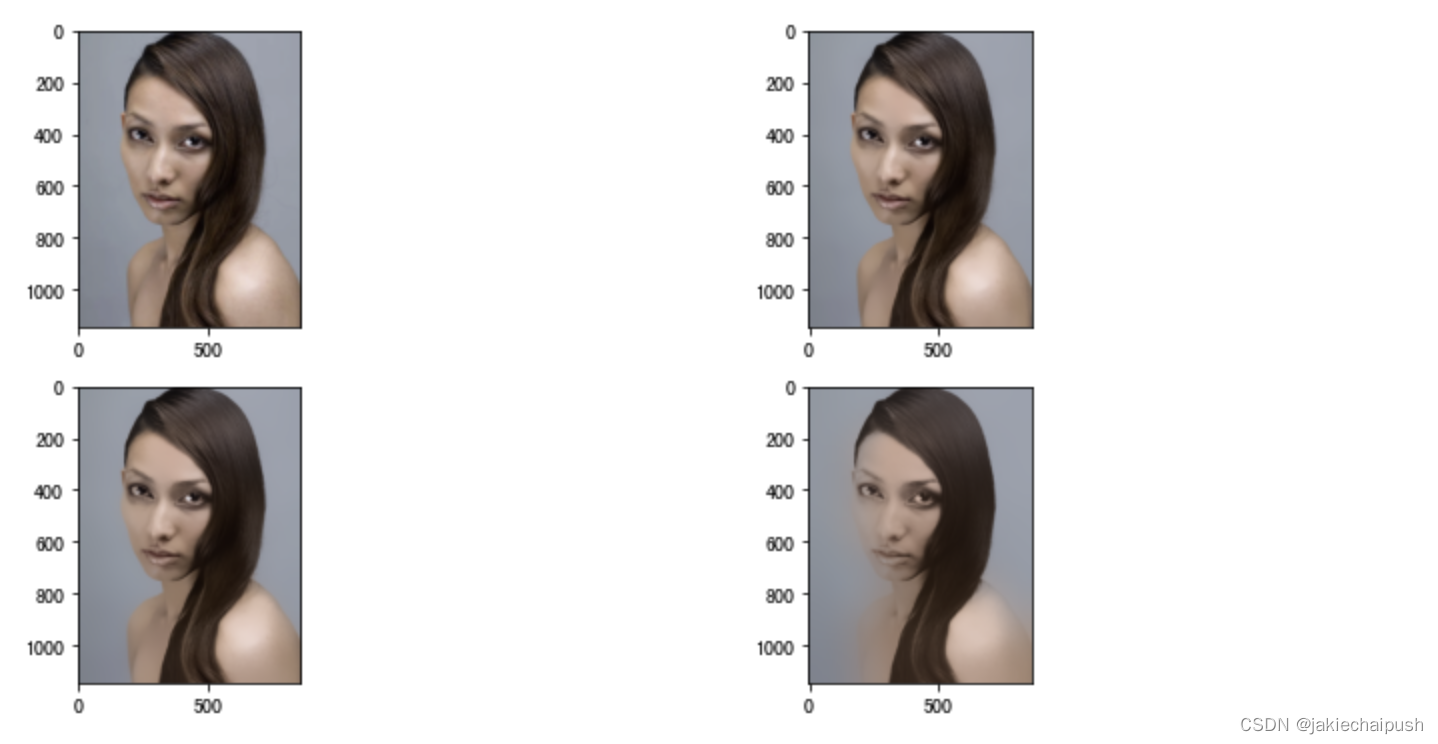# 一. Pillow处理图片

## 1.1 Pillow安装

`conda install pillow`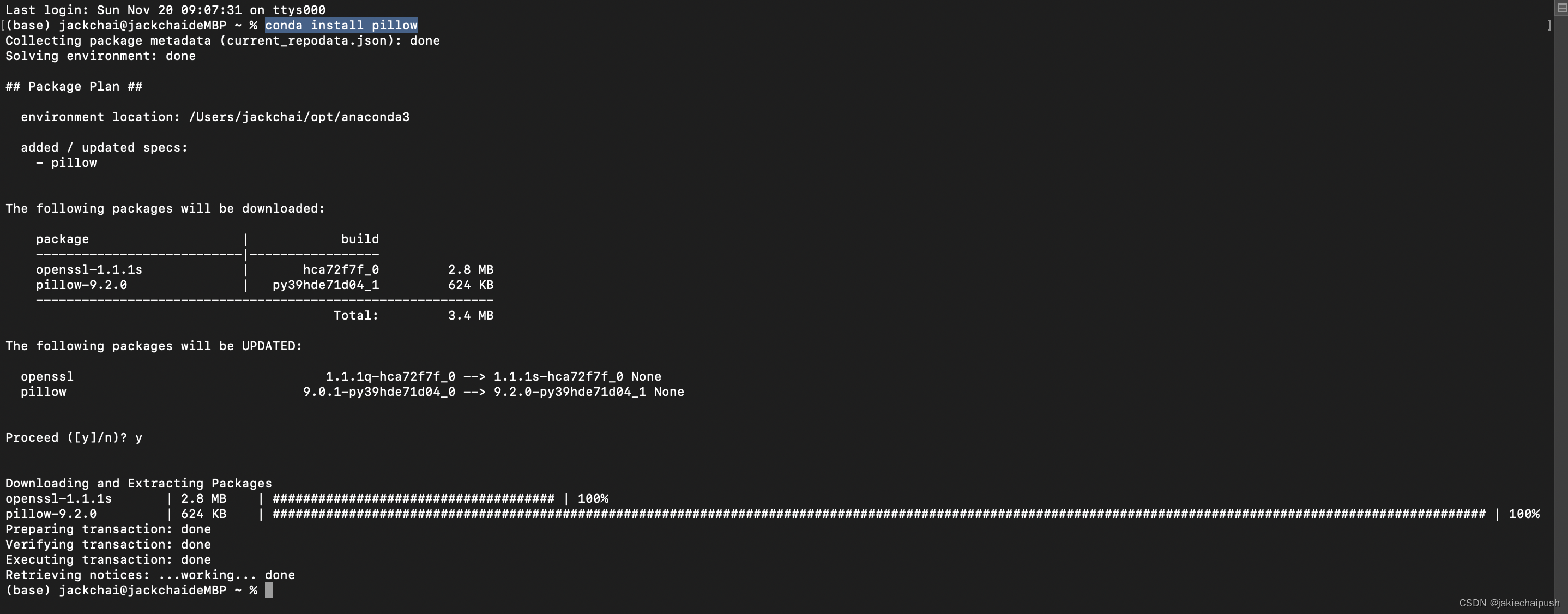## 1.2 Pillow基本使用

1. 读取并显示图片
```from PIL import Image  #导入图像库
cat=Image.open("./beauty.jpg")
print(type(cat))
cat```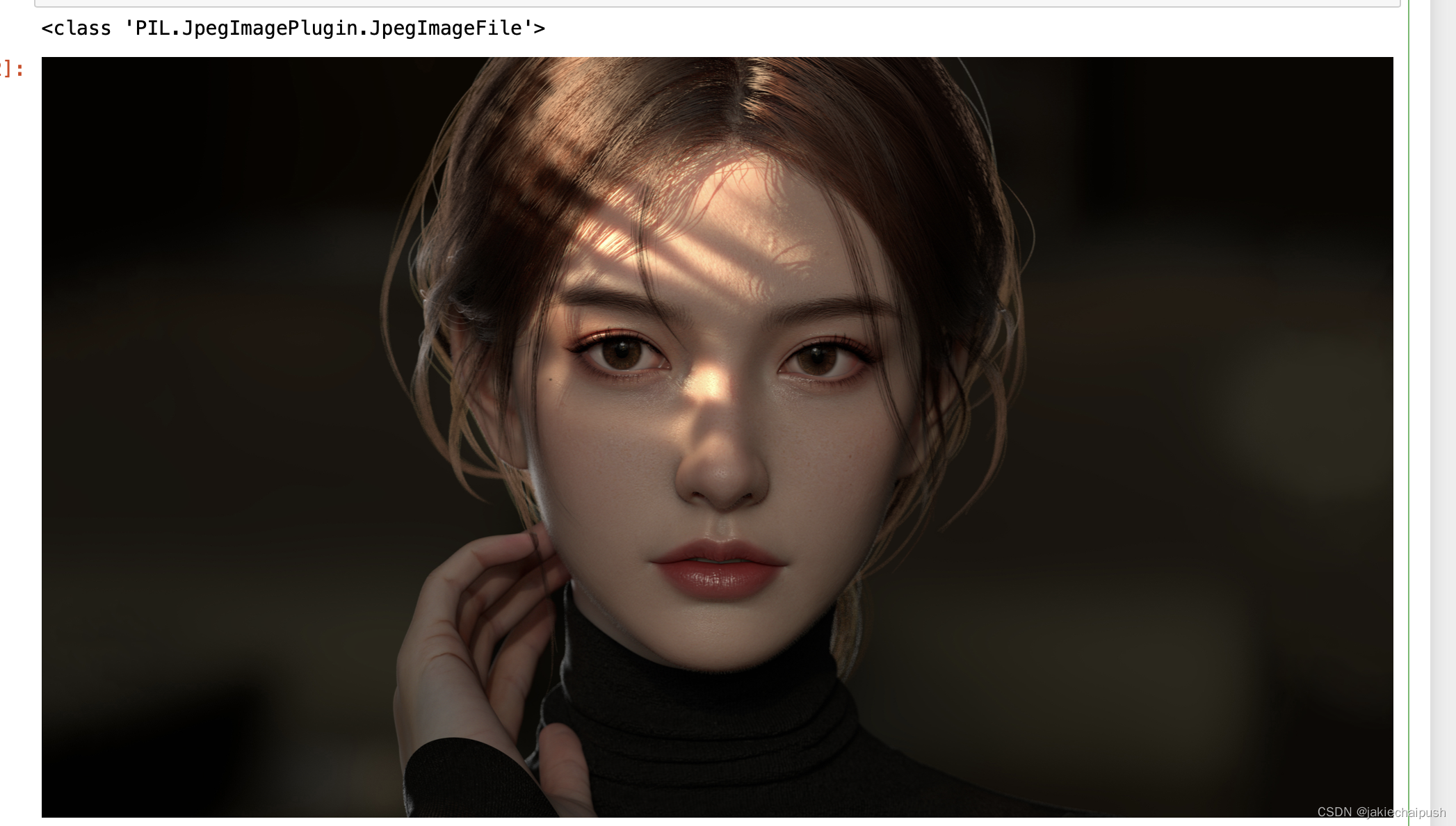2. 将图像数据转换为numpy数据

```from PIL import Image  #导入图像库
import numpy as np
beauty=Image.open("./beauty.jpg")
beauty_data=np.array(beauty)#图像转为ndarray对象
print(type(beauty_data))
print(beauty_data)```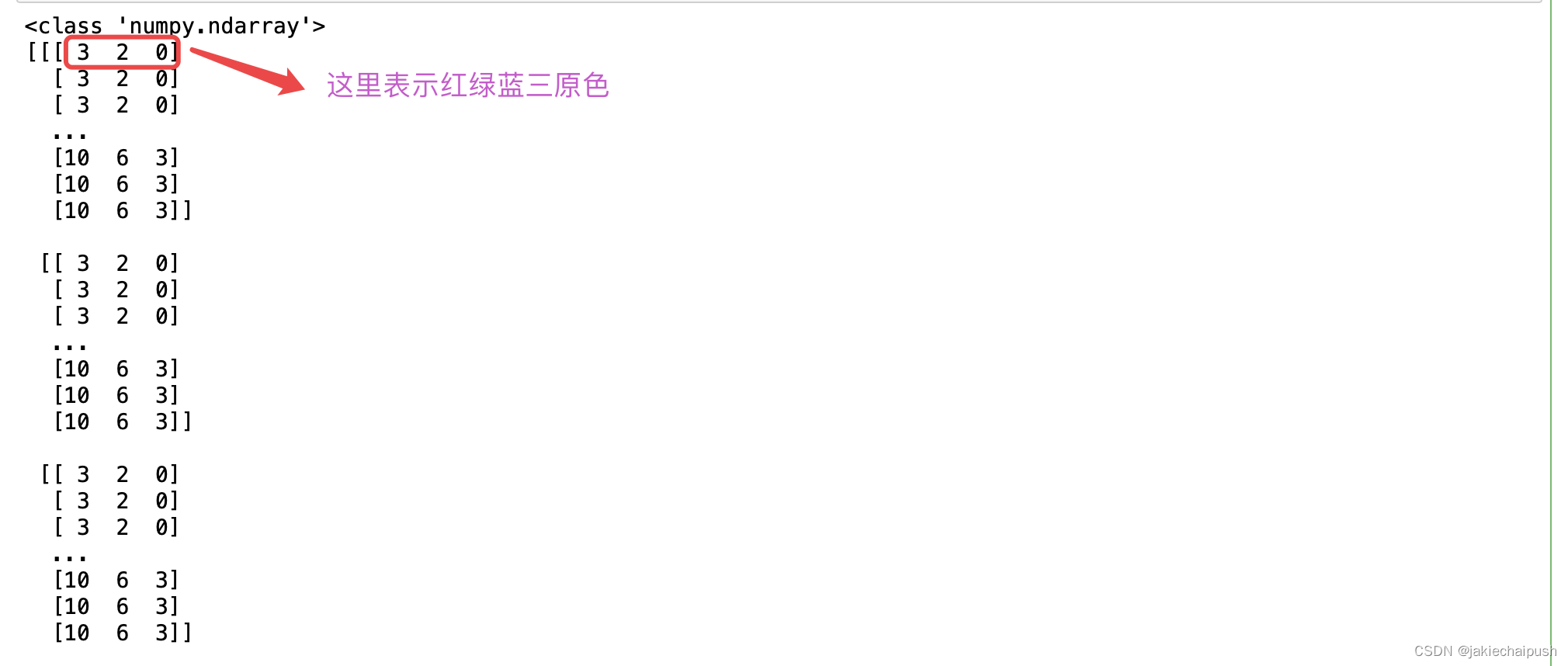3. 通过numpy对图像进行一些简单操作

```from PIL import Image  #导入图像库
import numpy as np
beauty=Image.open("./beauty.jpg")
beauty_data=np.array(beauty)
beauty_data=beauty_data[:,:,::-1] #这里将数据反向打印，所以红绿蓝变成了蓝绿红
beauty2=Image.fromarray(beauty_data) #将array数组转换为图片
beauty2```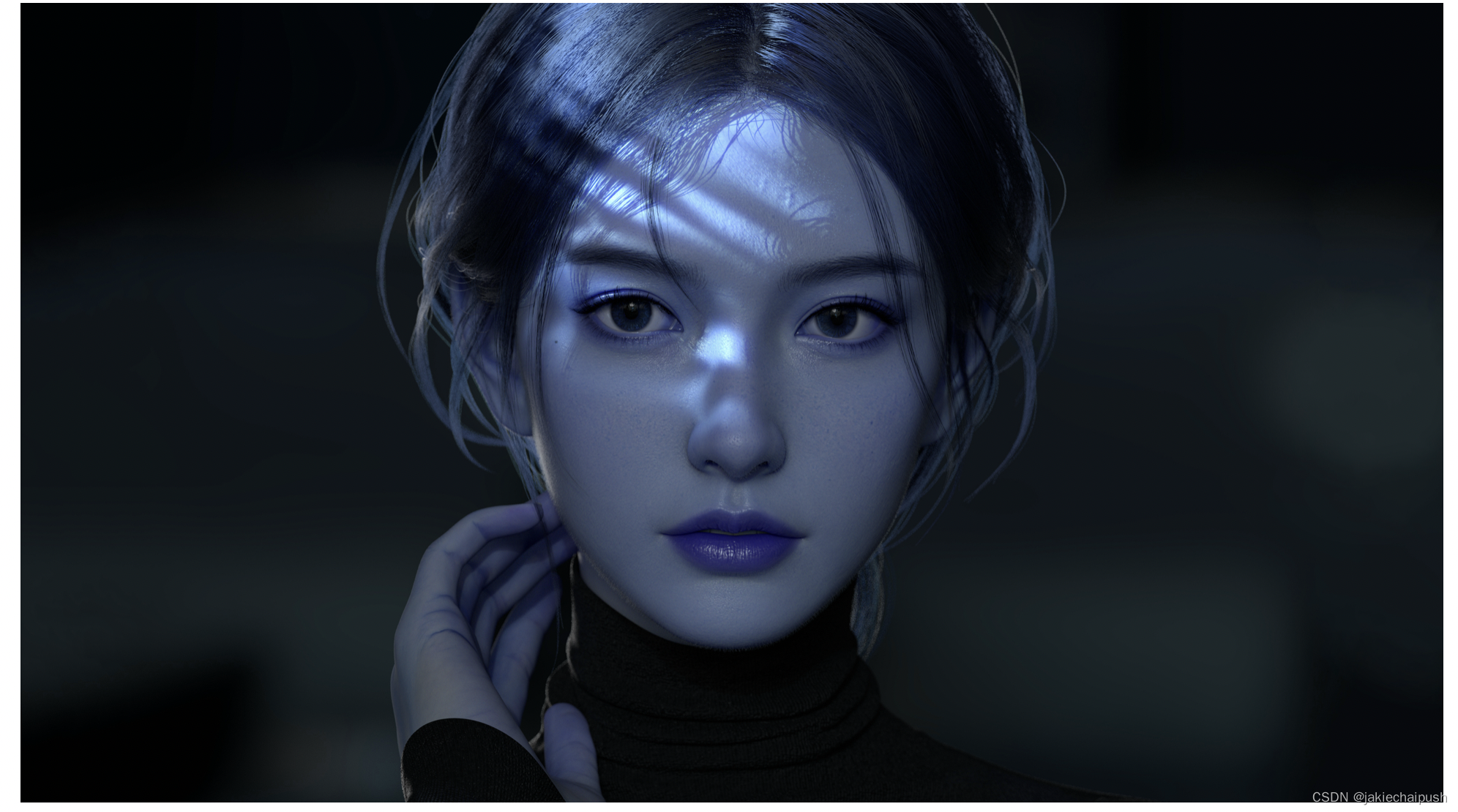# 二. Matplotlib包中的图像模块

## 2.1 简介

Matplotlib提供加载，重新缩放和显示图像所需的功能，但是Matplotlib仅支持PNG图像，如果本机读取失败，下面显示的命令将回腿到Pillow

Matplotlib已将每个通道的8位数据重新调整为0.0到1.0之间的浮点数据。Pillow可以使用的唯一数据类型是UTF8.Matplotlib绘图可以处理float32和uint8，但是除PNG之外的任何格式的图像读/写都限于uint8。为什么8位？大多数显示器每个通道只能渲染8位颜色等级。为什么他们只能渲染8位颜色等级？因为那是人眼能看到的。

## 2.2 API简介

```import numpy as np
import matplotlib.image as mpimg

print(type(data))
print(data)```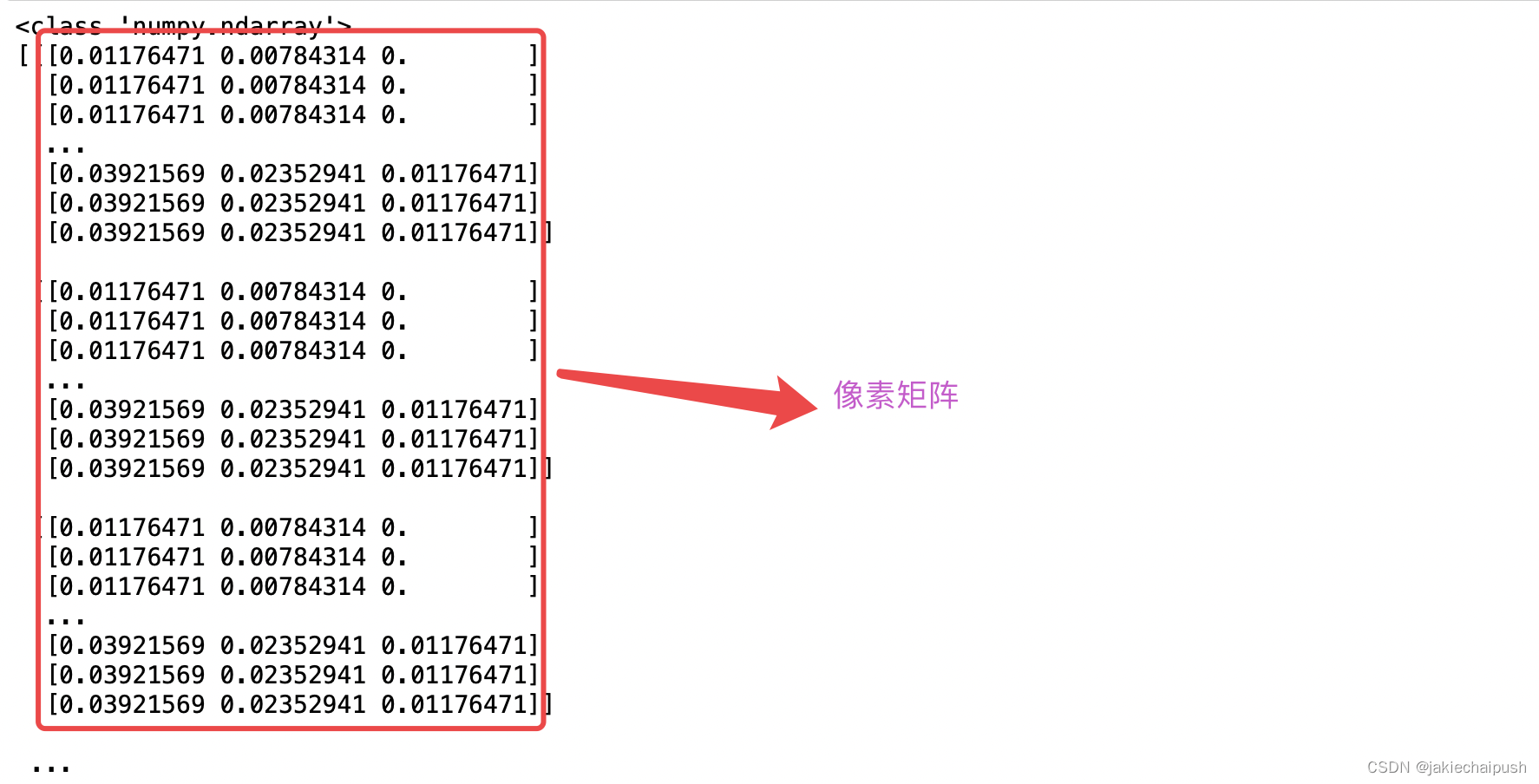imshow()

```import numpy as np
import matplotlib.image as mpimg
import matplotlib.pyplot as plt

plt.axis("off") #关闭网格
plt.imshow(data)```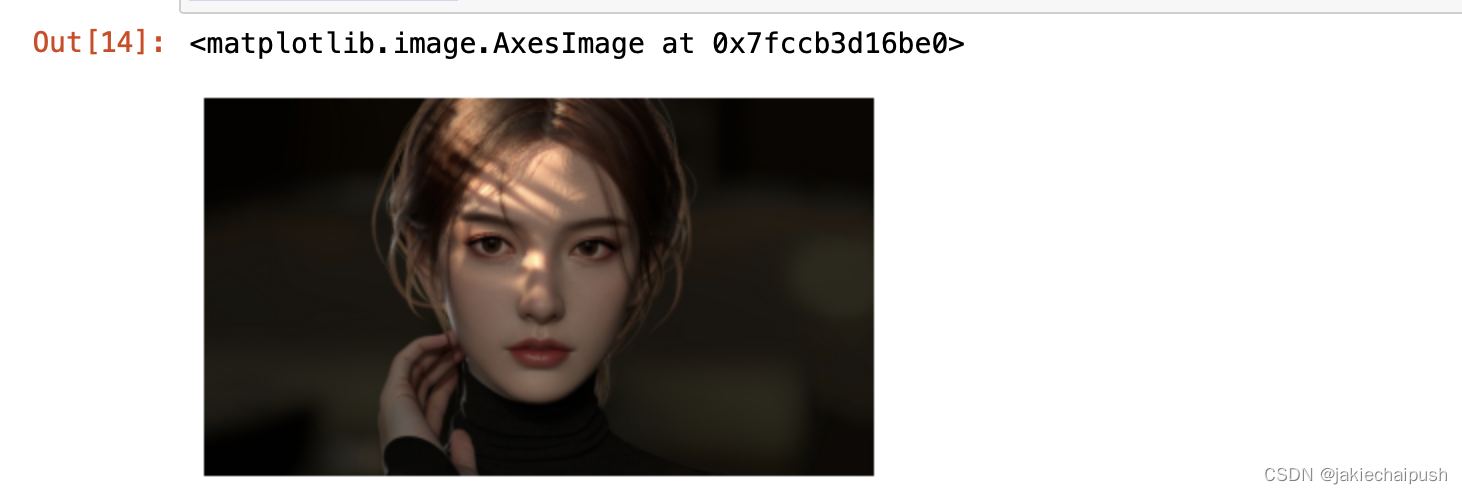imsave()

```import numpy as np
import matplotlib.image as mpimg
import matplotlib.pyplot as plt

plt.imsave("./beauty2.png",data,cmap="gray",origin="lower")#lower实现图像倒着显示```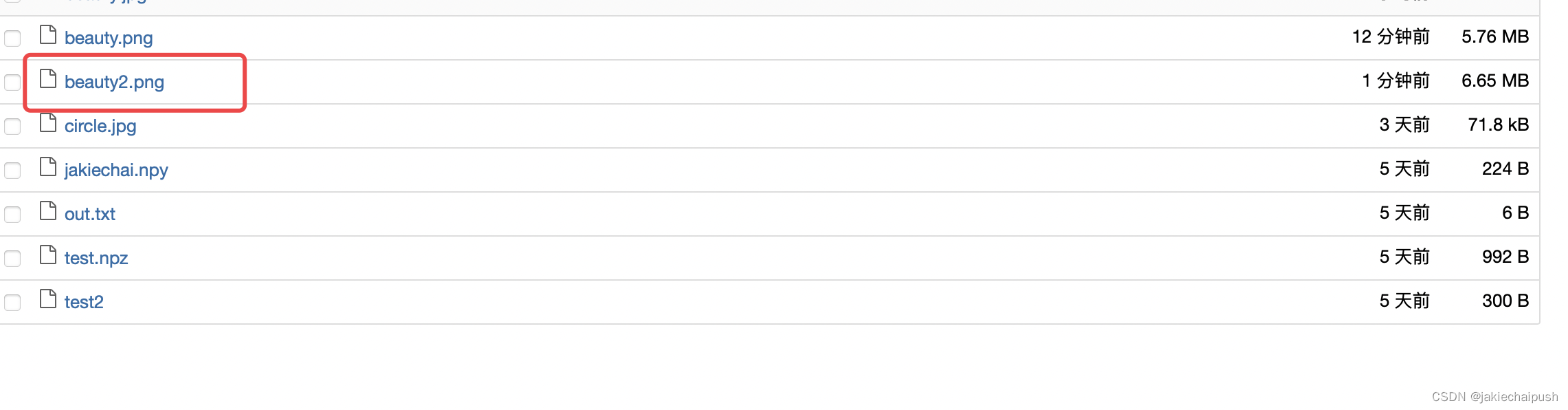# 三. ndarray图像操作练习

## 3.1 旋转图片

```import numpy as np
import matplotlib.image as mpimg
import matplotlib.pyplot as plt
plt.axis("off") #关闭轴线
data2=data[::,::-1,::]
plt.imshow(data2)```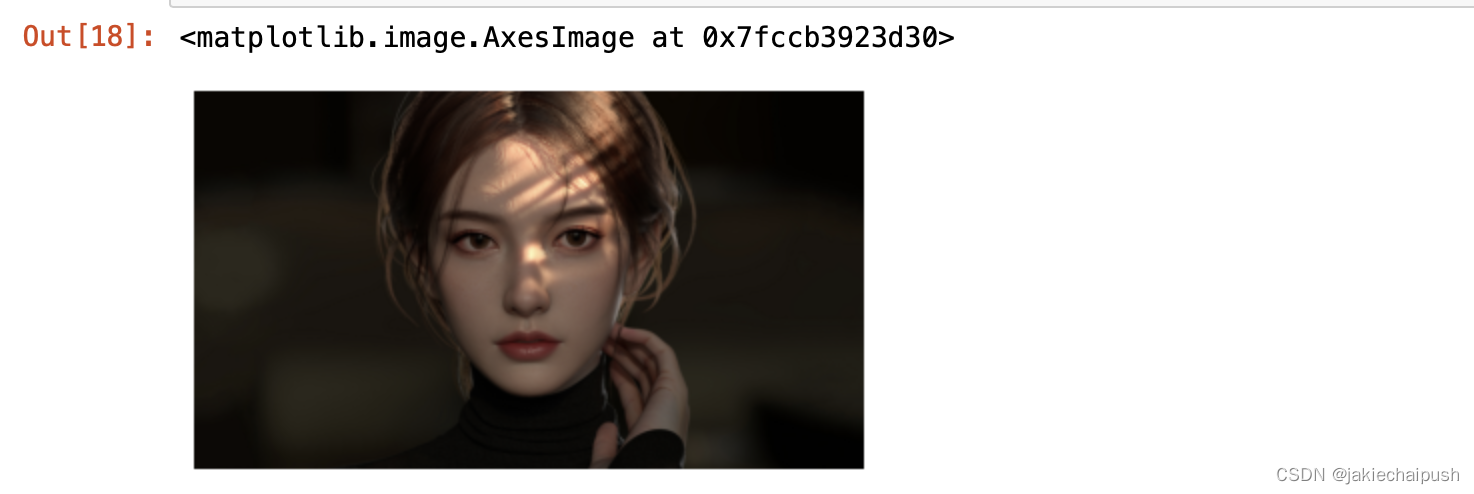## 3.2 图片打码

```import numpy as np
import matplotlib.image as mpimg
import matplotlib.pyplot as plt
plt.axis("off") #关闭轴线
data2=data[::80,::80] #step设置为20，只读了一部分数据
plt.imshow(data2)```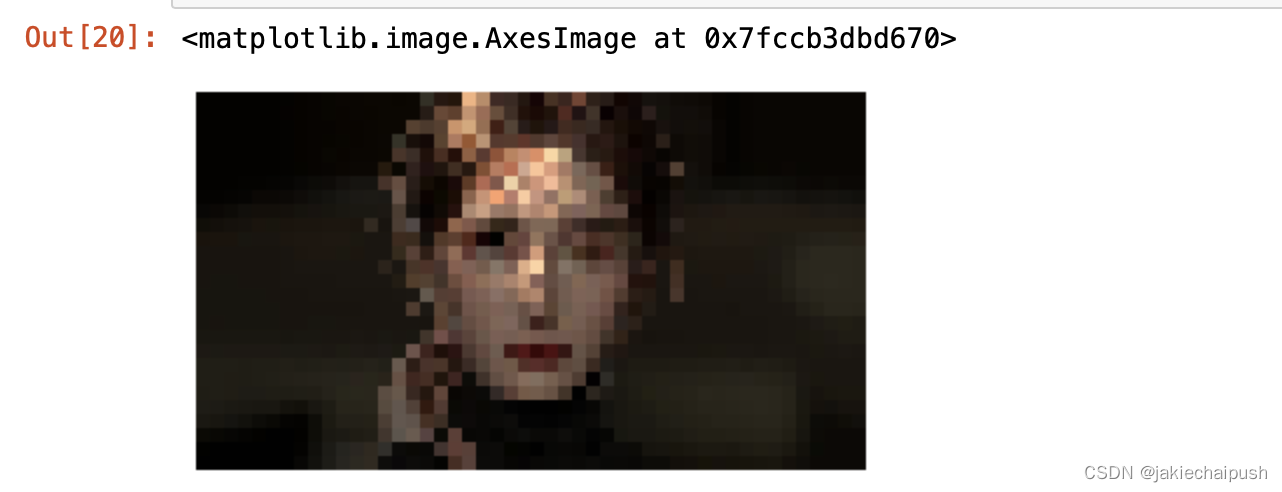1. 对图像进形裁剪，获得人脸区域
```import numpy as np
import matplotlib.image as mpimg
import matplotlib.pyplot as plt
plt.axis("off") #关闭轴线
data2=data[200:1800,1300:2500]
plt.imshow(data2)```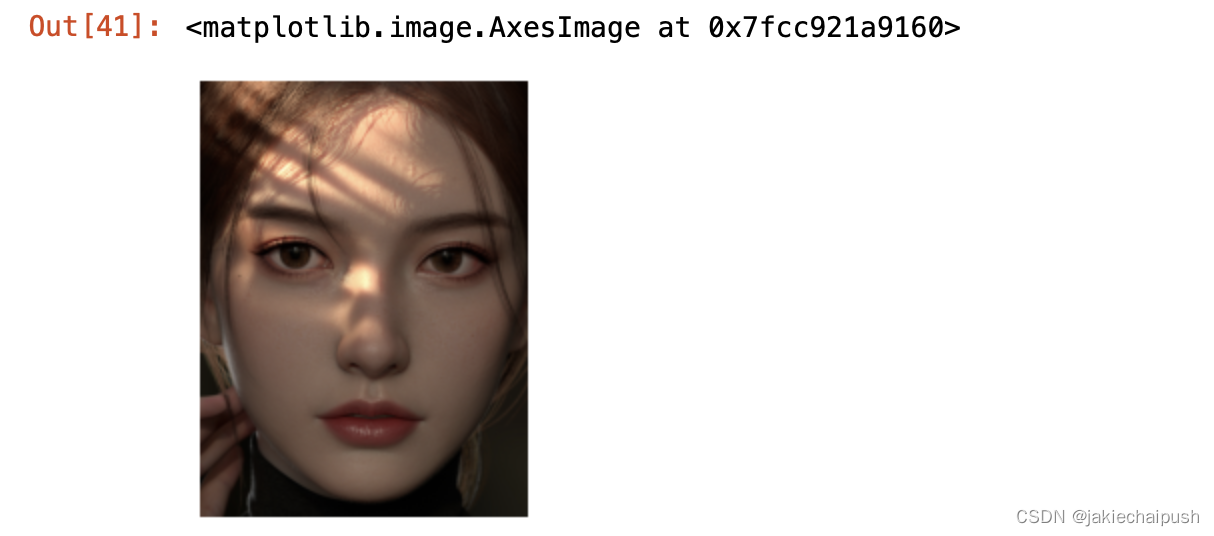2. 打码

```import numpy as np
import matplotlib.image as mpimg
import matplotlib.pyplot as plt
plt.axis("off") #关闭轴线
data2=data[200:1800:80,1300:2500:80]
for i in range(20):  #由于data2的维度和data被马赛克替换的那一块的维度大小不一样，所以不能直接将马赛克的脸嵌入
for j in range(15):
data[200+i*80:200+80+i*80,1300+j*80:1300+80+j*80]=data2[i,j]
plt.imshow(data)```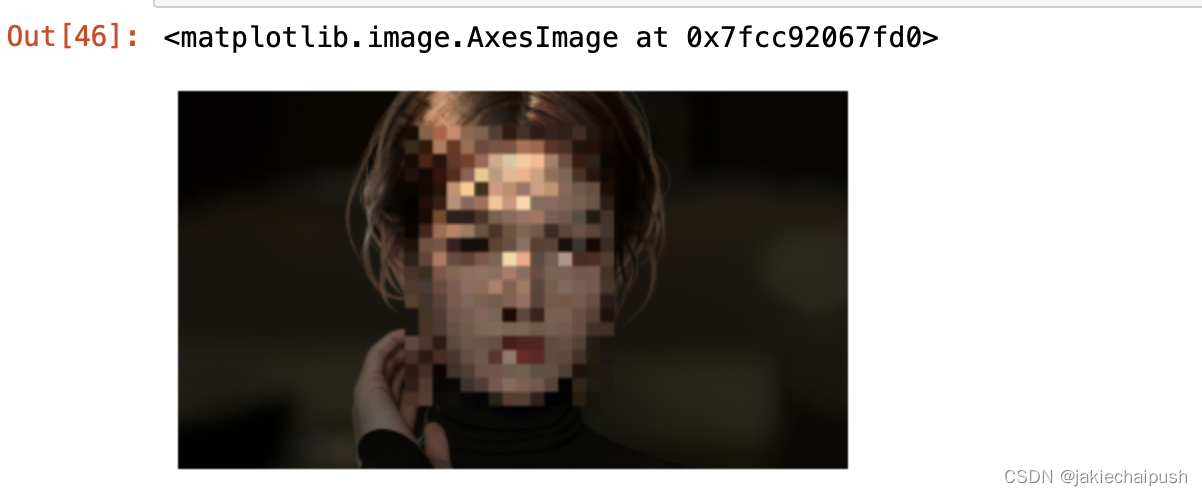## 3.3 图片拼接

```import numpy as np
import matplotlib.image as mpimg
import matplotlib.pyplot as plt
plt.axis("off") #关闭轴线
data3=np.concatenate([data1,data2],axis=1)
plt.imshow(data3)```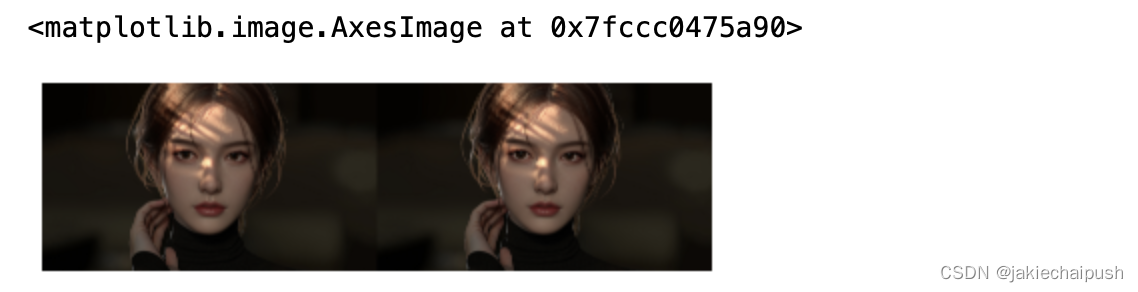## 3.4 图片切割

```import numpy as np
import matplotlib.image as mpimg
import matplotlib.pyplot as plt
plt.axis("off") #关闭轴线
d1,d2,d3=np.split(data1,indices_or_sections=[400,1800],axis=1) #切割大小为300，500
print(d1.shape,d2.shape,d3.shape)
plt.imshow(d2)```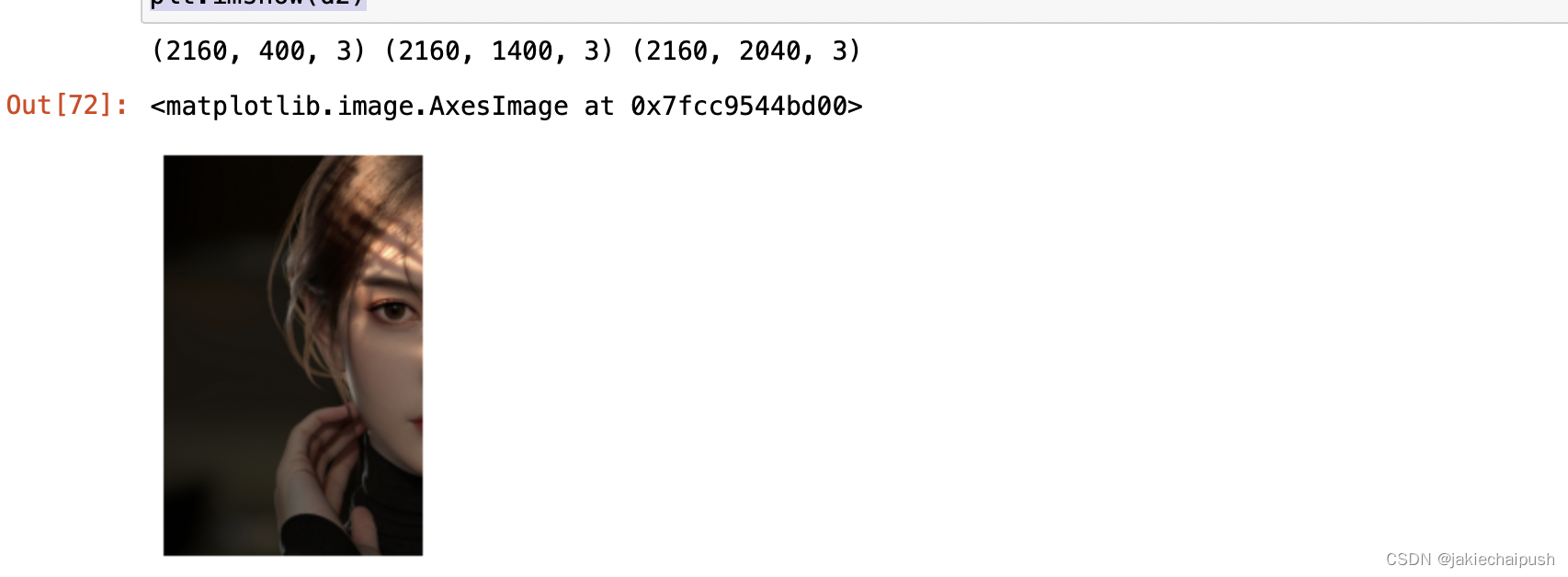# 四. 图像灰度化

## 4.2 灰度化方法

1. 最小值法或者是最大值法（极值法）

```import numpy as np
import matplotlib.image as mpimg
import matplotlib.pyplot as plt
plt.axis("off") #关闭轴线
data2=data1.min(axis=-1) #axis等于-1对应于最后一个维度，相当于取最后一个维度的最小值
plt.imshow(data2,cmap='gray')```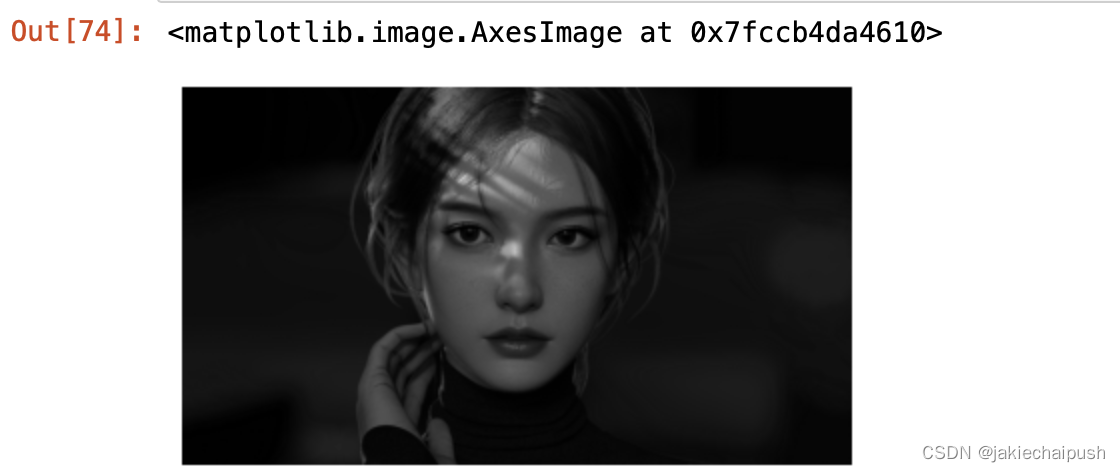```import numpy as np
import matplotlib.image as mpimg
import matplotlib.pyplot as plt
plt.axis("off") #关闭轴线
data2=data1.max(axis=-1) #axis等于-1对应于最后一个维度，相当于取最后一个维度的最小值
plt.imshow(data2,cmap='gray')```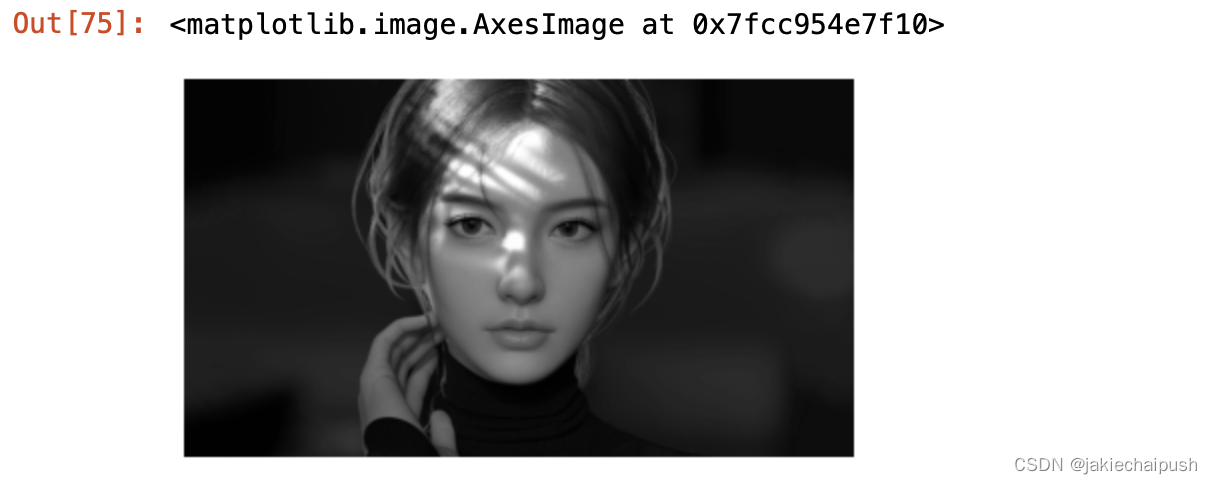2. 平均值法

```import numpy as np
import matplotlib.image as mpimg
import matplotlib.pyplot as plt
plt.axis("off") #关闭轴线
data2=data1.mean(axis=-1) #axis等于-1对应于最后一个维度，相当于取最后一个维度的最小值
plt.imshow(data2,cmap='gray')```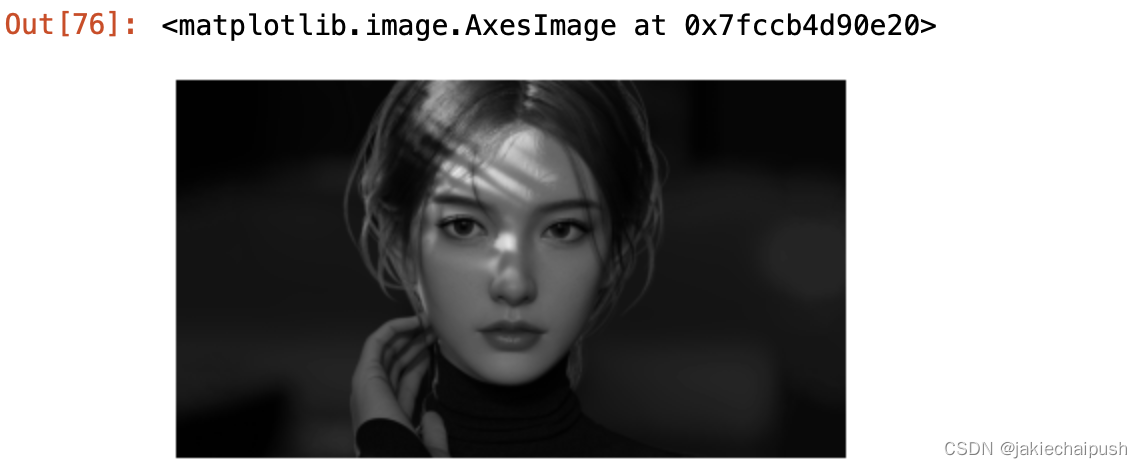3. 加权平均值法

```import numpy as np
import matplotlib.image as mpimg
import matplotlib.pyplot as plt
plt.axis("off") #关闭轴线
weight=[0.299,0.587,0.114]
data2=np.dot(data1,weight)
plt.imshow(data2,cmap='gray')```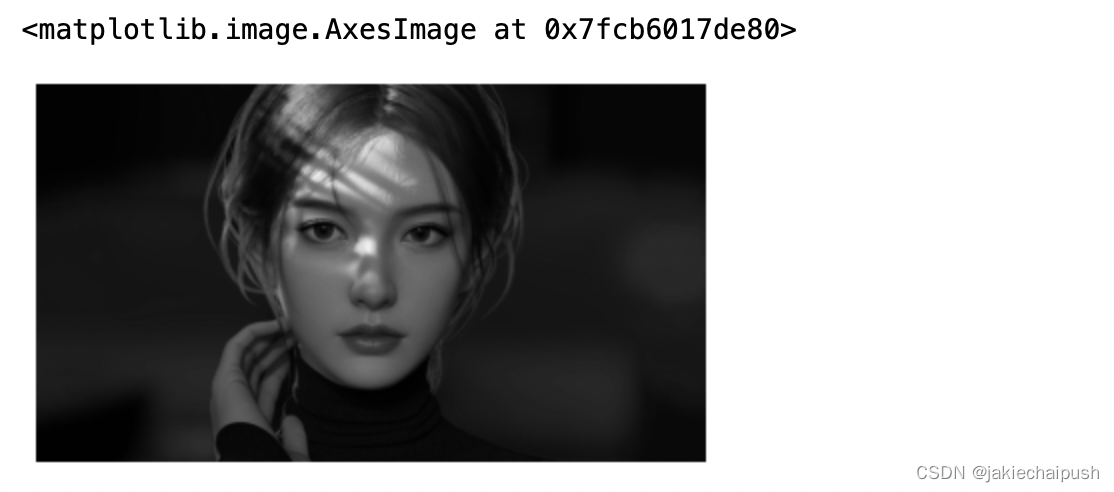# 五. OpenCV使用入门

## 5.1 简介

OpenCV是一个基于Apache2.0许可（开源）发行的跨平台计算机视觉和机器学习软件库，可以运行在Linux、Windows、AndroidMac OS操作系统上。  它轻量级而且高效——由一系列 C 函数和少量 C++ 类构成，同时提供了Python、Ruby、MATLAB等语言的接口，实现了图像处理和计算机视觉方面的很多通用算法。OpenCV用C++语言编写，它具有C ++，Python，Java和MATLAB接口，并支持Windows，Linux，Android和Mac OS，OpenCV主要倾向于实时视觉应用，并在可用时利用MMX和SSE指令， 如今也提供对于C#、Ch、Ruby，GO的支持。opencv的下载命令：

`pip install opencv-python`

## 5.2 OpenCV基本使用

1. 读取图像
```import matplotlib.pyplot as plt
import cv2
plt.imshow(data)```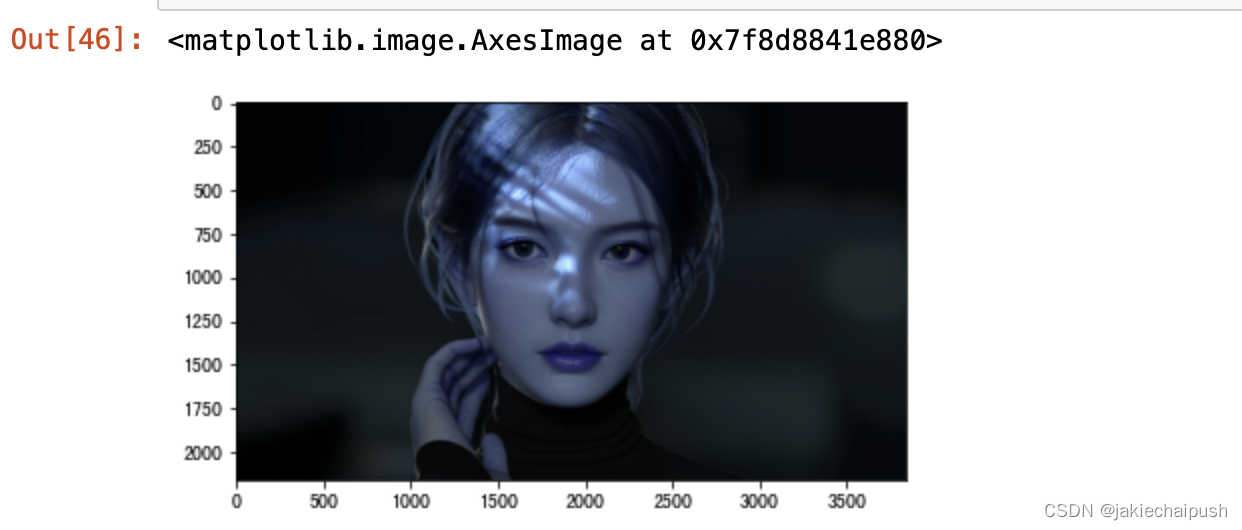```import matplotlib.pyplot as plt
import cv2
b,g,r=cv2.split(data)
data2=cv2.merge([r,g,b])
plt.imshow(data2)```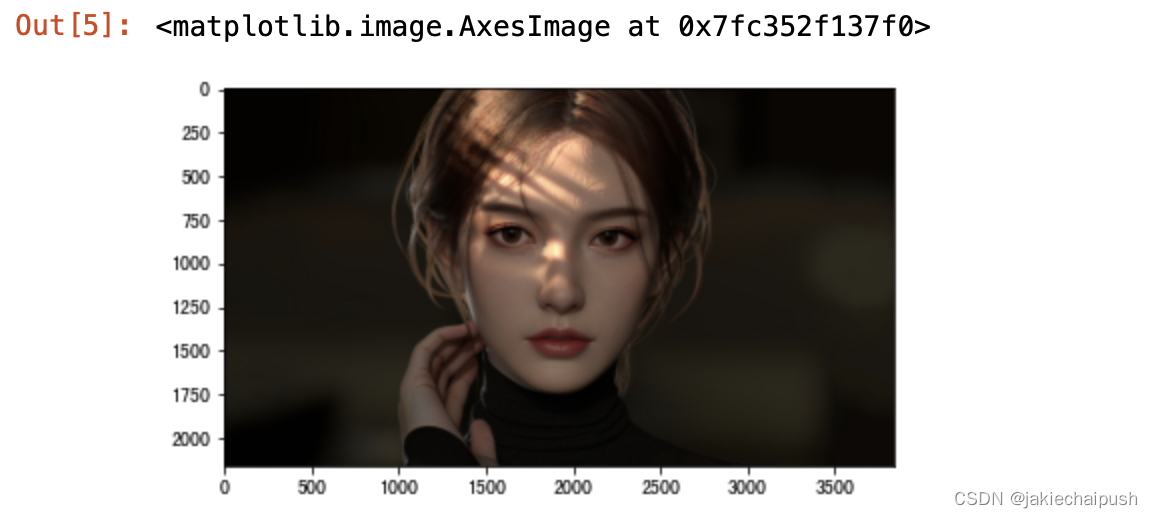2. 九种常见的颜色空间，包括BGR、RGB、GRAY、HSV、YCrCb、HLS、XYZ、LAB、YUV展示

BRG转RGB颜色空间

```import matplotlib.pyplot as plt
import cv2
#BGR->RGB 上面也提供了一种常见的转换形式
img_RGB=cv2.cvtColor(img_BGR,cv2.COLOR_BGR2RGB)
plt.imshow(img_RGB)```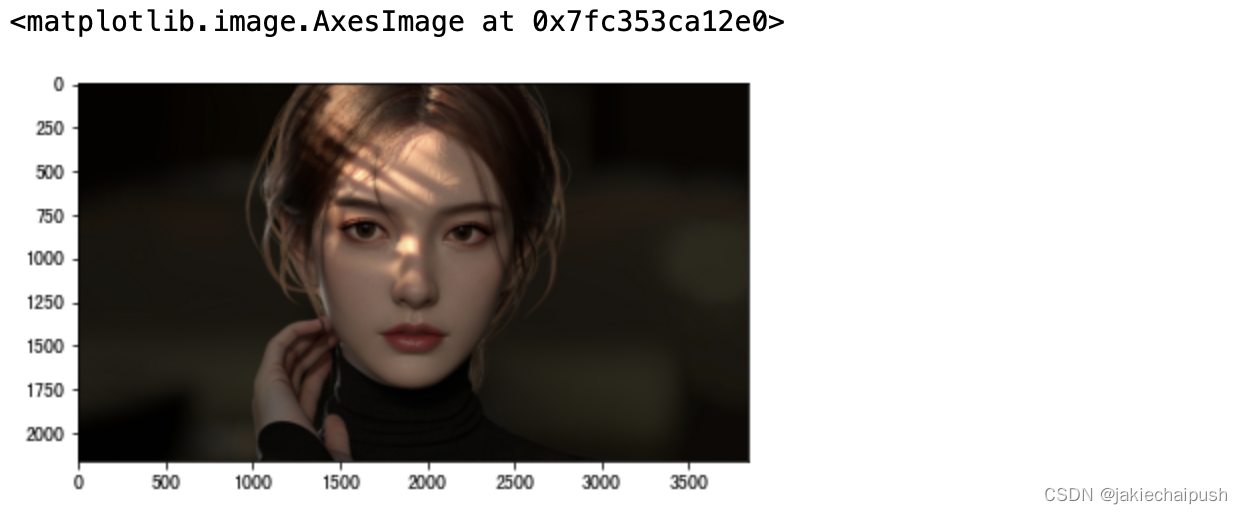```import matplotlib.pyplot as plt
import cv2
#BGR->RGB 上面也提供了一种常见的转换形式
img_RGB=cv2.cvtColor(img_BGR,cv2.COLOR_BGR2GRAY)
plt.imshow(img_RGB)``````image_HSV=cv2.cvtColor(ima_BGR,cv2.COLOR_BGR2HSV)
image_YCrCb=cv2.cvtColor(ima_BGR,cv2.COLOR_BGR2YCrCb)
image_HLS=cv2.cvtColor(ima_BGR,cv2.COLOR_BGR2HLS)
image_XYZ=cv2.cvtColor(ima_BGR,cv2.COLOR_BGR2XYZ)
image_LAB=cv2.cvtColor(ima_BGR,cv2.COLOR_BGR2LAB)
image_YUV=cv2.cvtColor(ima_BGR,cv2.COLOR_BGR2YUV)```

## 5.3 图像灰度化

1. 直接获取
```import matplotlib.pyplot as plt
import cv2
#BGR->RGB 上面也提供了一种常见的转换形式
img_RGB=cv2.cvtColor(img_BGR,cv2.COLOR_BGR2GRAY)
plt.imshow(img_RGB,'gray')```2. 同意也可以使用 4.2中介绍的灰度化方法（这里我们使用最小值法）

```import matplotlib.pyplot as plt
import cv2
import numpy as np
#BGR->RGB 上面也提供了一种常见的转换形式
img_RGB=cv2.cvtColor(img_BGR,cv2.COLOR_BGR2RGB)
row,col,channel=img_RGB.shape
for i in range(row):
for j in range(col):
img_RGB[i,j]=np.uint8(min(img_RGB[i,j,0],img_RGB[i,j,1],img_RGB[i,j,2]))
plt.imshow(img_RGB)```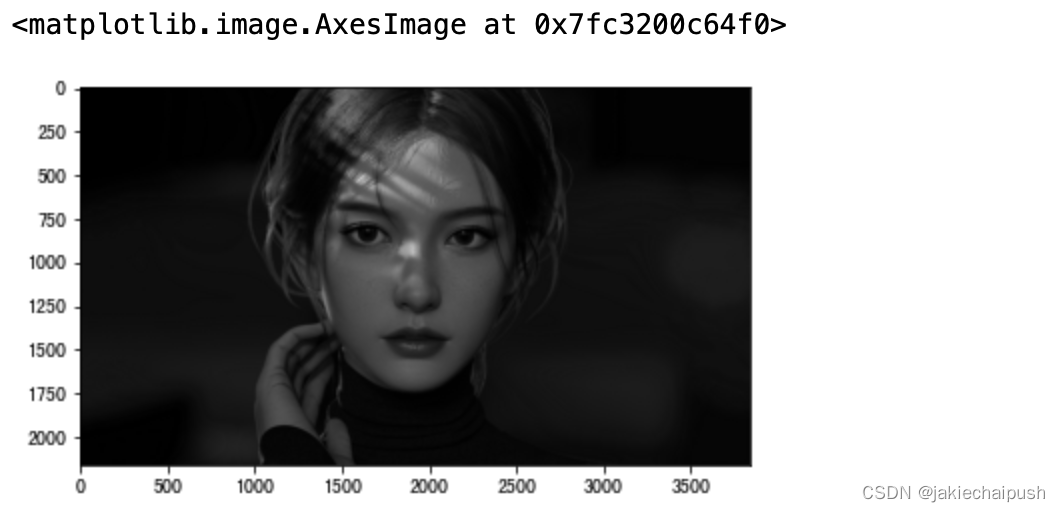## 5.4 图像二值化

src 原图像
thresh 设定阈值
maxval 高于（低于）阈值时所赋予的像素新值
type 选择参数
type值 说明
cv2.THRESH_BINARY 黑白二值
cv2.THRESH_BINARY_INV 黑白二值反转
cv2.THRESH_TRUNC 得到的图像为多像素
cv2.THRESH_TOZERO
cv2.THRESH_TOZERO_INV

```import matplotlib.pyplot as plt
import cv2
data=cv2.cvtColor(data,cv2.COLOR_BGR2GRAY) #二值化之前要将图片转换为灰度图片
ret1,thresh1=cv2.threshold(data,42,255,cv2.THRESH_BINARY)#大于阈值设置为255，小于阈值设置为0，设置合适的阈值才有号的效果
ret2,thresh2=cv2.threshold(data,42,255,cv2.THRESH_BINARY_INV)
ret3,thresh3=cv2.threshold(data,42,255,cv2.THRESH_TRUNC)
ret4,thresh4=cv2.threshold(data,42,255,cv2.THRESH_TOZERO)
ret5,thresh5=cv2.threshold(data,42,255,cv2.THRESH_TOZERO_INV)
titles=['img','THRESH_BINARY','THRESH_BINARY_INV',"THRESH_TRUNC",'THRESH_TOZERO','THRESH_TOZERO_INV']
images=[data,thresh1,thresh2,thresh3,thresh4,thresh5]
for i in range(6):
plt.subplot(2,3,i+1)
plt.imshow(images[i],'gray')
plt.title(titles[i])
plt.xticks([])
plt.yticks([])
plt.show()```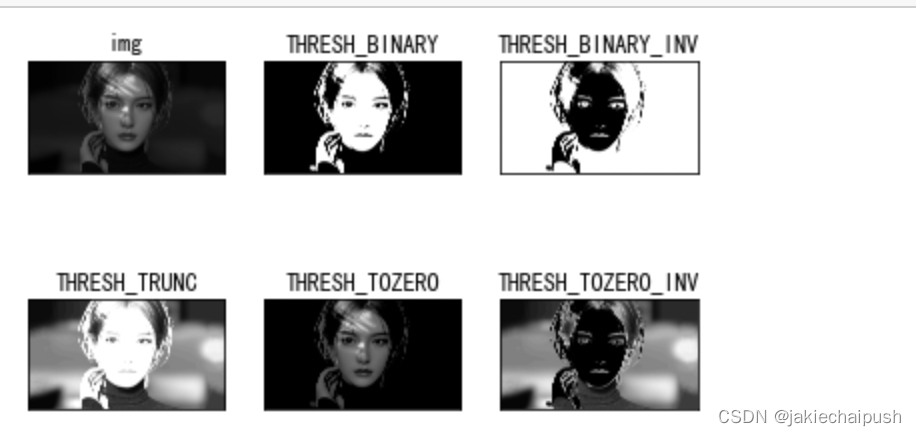src 原图像
maxValue 高于（低于）阈值时赋予的新值
thresholdType 赋值方法，只有cv2.THRESH_BINARY和cv2.THRESH_BINARY_INV
blockSize 规定领域的大小(一个正方形区域)
C 常数，阈值等于均值或者加权值减去这个常数（为0相当于阈值就是求得领域内均值或者加权值）
```import matplotlib.pyplot as plt
import cv2
data=cv2.cvtColor(data,cv2.COLOR_BGR2GRAY) #二值化之前要将图片转换为灰度图片
images=[data,th2,th3]
plt.figure()
for i in range(3):
plt.subplot(1,3,i+1)
plt.imshow(images[i],'gray')
plt.show()```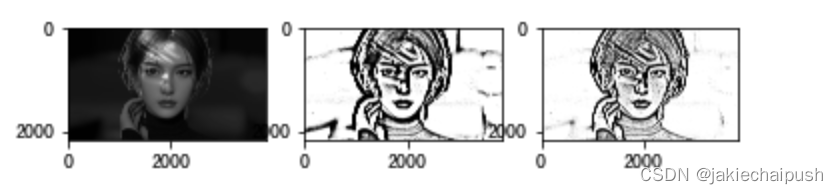Otsu`s二值化
cv2.threshold()函数是有两个返回值的，前面一直用的第二个返回值，也就是阈值处理后的图像，那么第一个返回值（得到图像的阈值）这里将会用到。前面对于阈值的处理上，我们选择阈值为42，在实际情况下，42其实并不是最好的阈值 ，那么怎么去找最好的阈值？我们需要算法去自己去寻找一个阈值，而Otsu`s就可以自己加找到一个认为最好的阈值，并且Otsu`s非常适合于图像灰度直方图具有双峰的情况，他会在双峰之间找到一个值作为阈值，对于双峰图像，可能并不是最好用，那么经过Otsu`s得到的那个阈值就是函数cv2.threshold的第一个参数了。因为Otsu`s方法会产生一个阈值，那么函数cv2.threshold的第二个参数（设置阈值）就是0了，并且在cv2.threshold的方法参数中还得加上语句cv2.THRESH_OTSU

```import matplotlib.pyplot as plt
import cv2
data=cv2.cvtColor(data,cv2.COLOR_BGR2GRAY) #二值化之前要将图片转换为灰度图片
ret1 ,th1=cv2.threshold(data,42,255,cv2.THRESH_BINARY)
ret2,th2=cv2.threshold(data,0,255,cv2.THRESH_BINARY+cv2.THRESH_OTSU)
print(ret2)```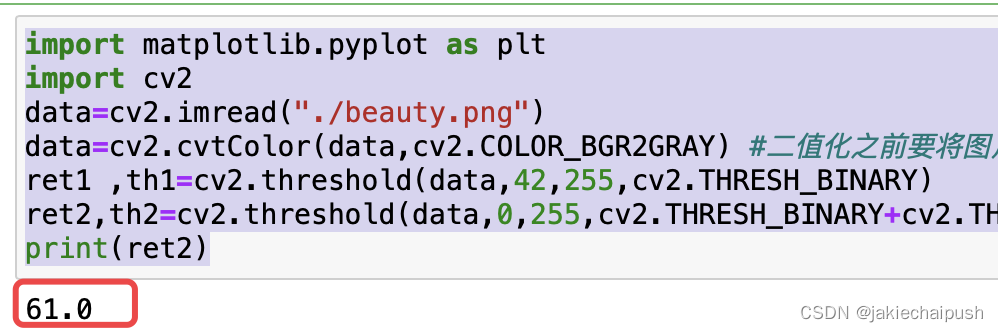```images=[data,th1,th2]
plt.figure()
for i in range(3):
plt.subplot(1,3,i+1)
plt.imshow(images[i],'gray')
plt.show()```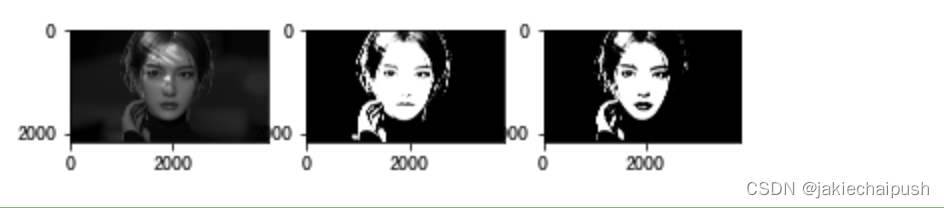```plt.figure()
plt.hist(data.ravel(),256)
plt.show()```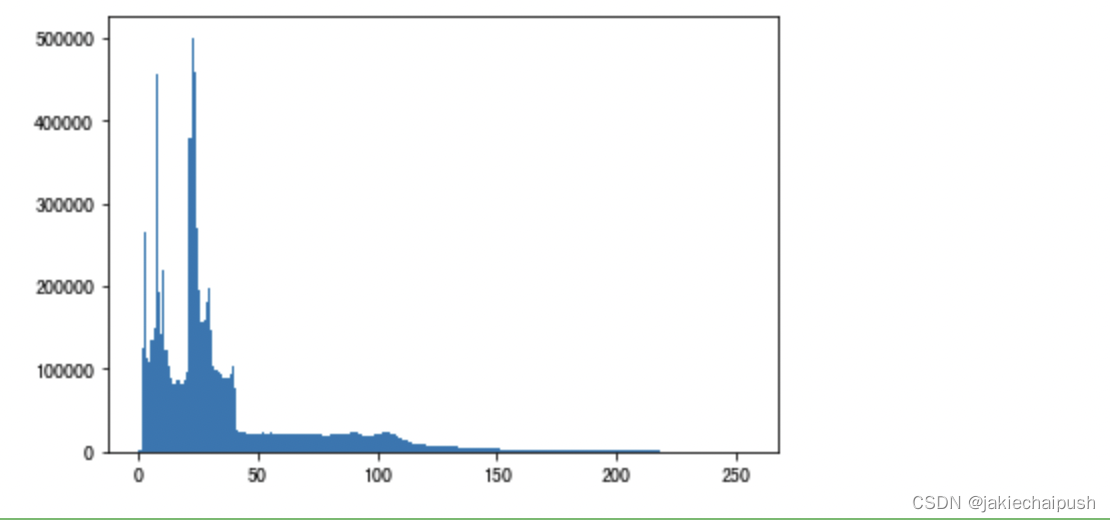## 5.5 图像降噪

1. 均值滤波
概念：个KxK大小的窗口从图像左上角开始，从左到右，从上到下划过整个图像，这个KxK的窗口称为卷积核，卷积核的锚点一般位于窗口的中心位置，所以卷积核的边长一股为奇数。在滑窗的过程中，计算窗口内像素的平均值，用这个平均值来代替锚点的像素值，遍历过后，图像的纹理信息减弱，噪声减弱，图像变得平滑
特点：Ksize越到，图像越平滑，也就是越模糊，是线性滤波器
函数：res=cv2.blur(image,(ksize,ksize))
作用：可以作用RGB图像
```import matplotlib.pyplot as plt
import cv2
data=cv2.cvtColor(data,cv2.COLOR_BGR2RGB)
#均值滤波
data2=cv2.blur(data,(9,9))#9x9卷积核大小
data3=cv2.blur(data,(19,19))
data4=cv2.blur(data,(100,100))
images=[data,data2,data3,data4]
for i in range(4):
plt.subplot(2,2,i+1)
plt.imshow(images[i],'gray')
plt.show()#可以看到卷积核越大图片越模糊```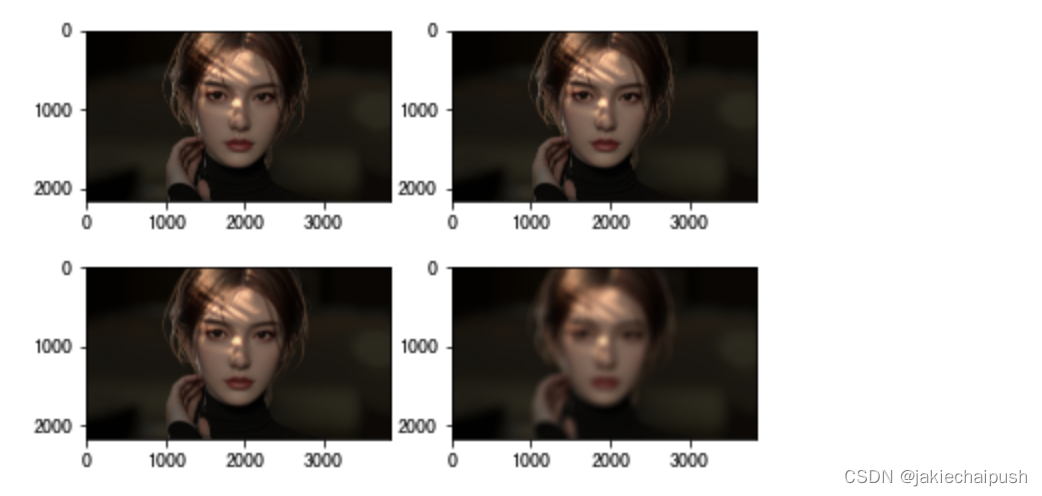2. 高斯滤波

res=cv2.GaussianBlur(image_data, (size, size), sigmaX, sigmaY)

```import matplotlib.pyplot as plt
import cv2
data=cv2.cvtColor(data,cv2.COLOR_BGR2RGB)
#均值滤波
data2=cv2.GaussianBlur(data,(9,9),0)#9x9卷积核大小
data3=cv2.GaussianBlur(data,(51,51),0)
data4=cv2.GaussianBlur(data,(101,101),0)
images=[data,data2,data3,data4]
for i in range(4):
plt.subplot(2,2,i+1)
plt.imshow(images[i],'gray')
plt.show()#可以看到卷积核越大图片越模糊```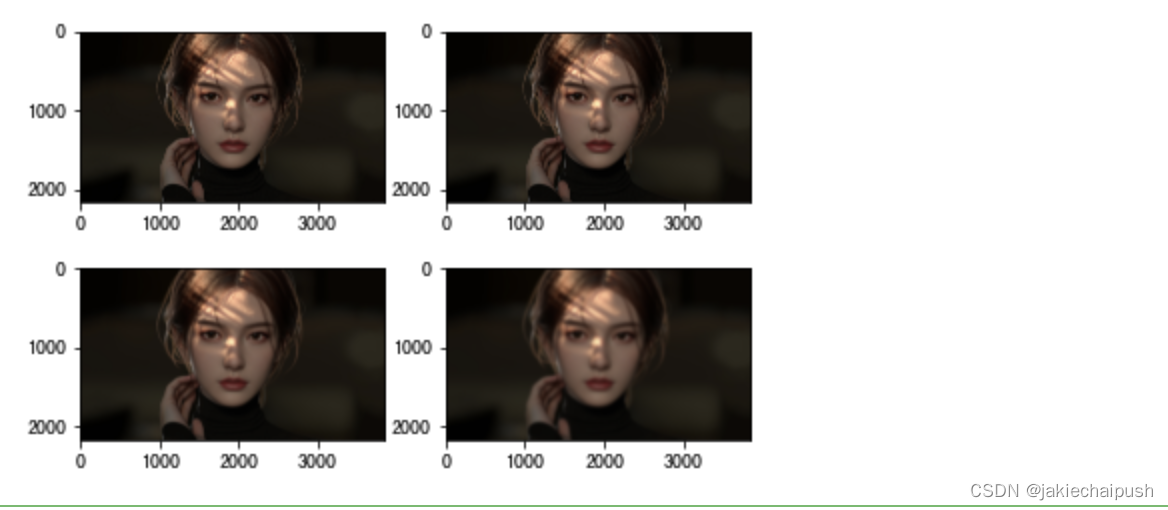3. 中值滤波

tip：椒盐噪声分为椒噪声(pepper)和盐噪声(salt)。椒嗓声点处灰度值为0，盐嗓声点处灰度值为255

```import numpy as np
def make_sp_noise(image,ratio):
"""人为的为图像添加椒盐噪声，ratia规定了噪声点占全局像素的比例"""
h,w=image.shape[:2]
image_copy=image.copy()
nums=int(h*w*ratio) #椒盐噪声点占比
for i in range(nums):
row=np.random.randint(0,h)
col=np.random.randint(0,w)
if i%2==0:
image_copy[row,col]=255
else:
image_copy[row,col]=0
return image_copy```

```import matplotlib.pyplot as plt
import cv2
data=cv2.cvtColor(data,cv2.COLOR_BGR2RGB)
data2=make_sp_noise(data,0.6)#添加椒盐噪音
plt.imshow(data2)```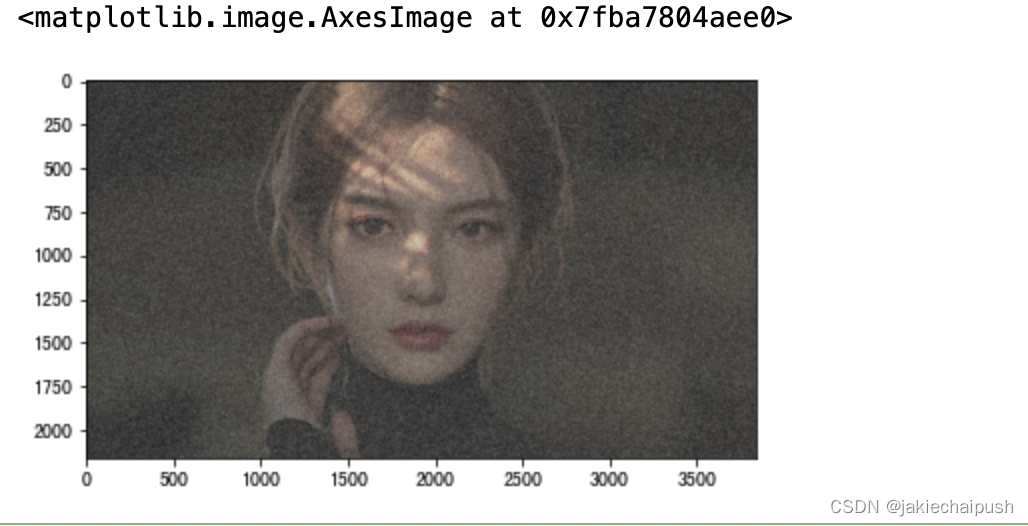```import matplotlib.pyplot as plt
import cv2
data=cv2.cvtColor(data,cv2.COLOR_BGR2RGB)
data=make_sp_noise(data,0.6)
data2=cv2.medianBlur(data,1)#9x9卷积核大小
data3=cv2.medianBlur(data,3)
data4=cv2.medianBlur(data,5)
plt.figure(figsize=(12,6))
images=[data,data2,data3,data4]
for i in range(4):
plt.subplot(2,2,i+1)
plt.imshow(images[i],'gray')
plt.show()```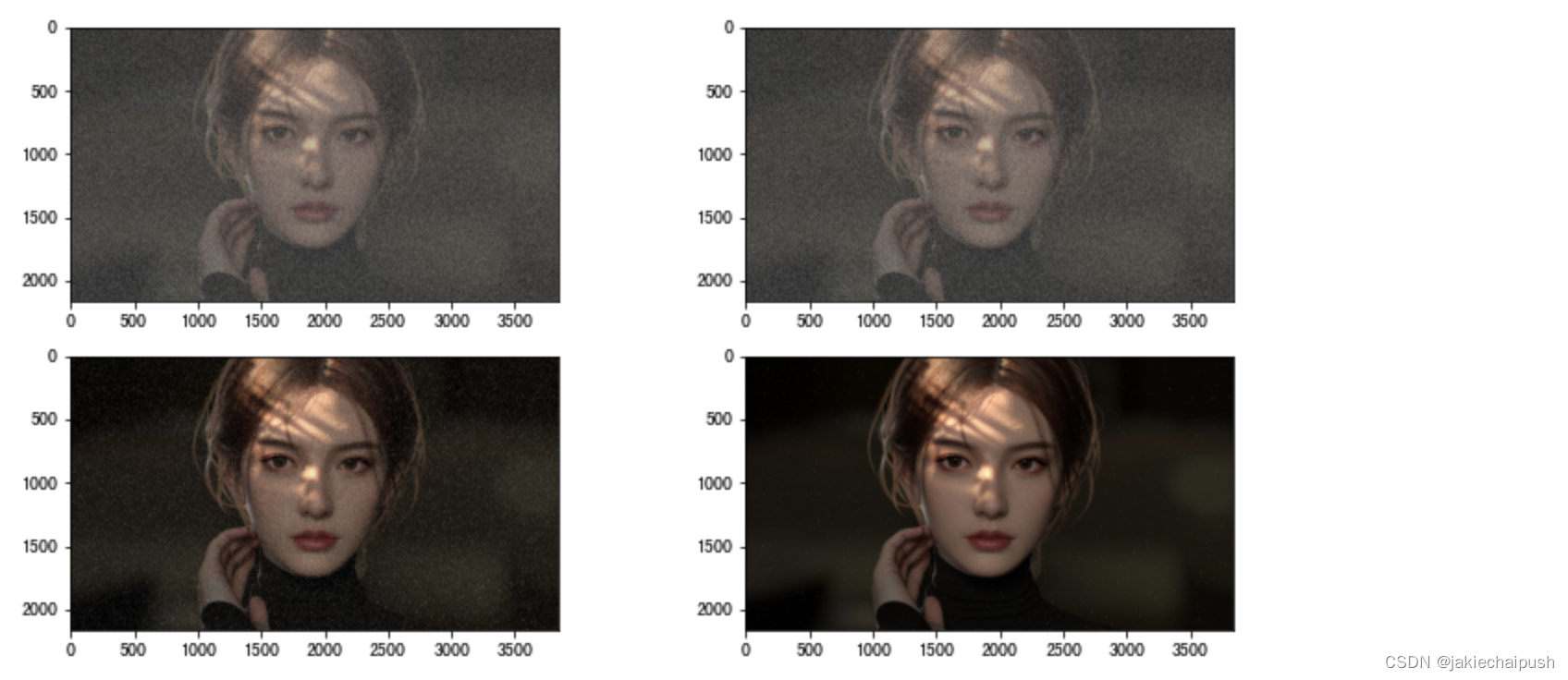4. 双边滤波

```import matplotlib.pyplot as plt
import cv2